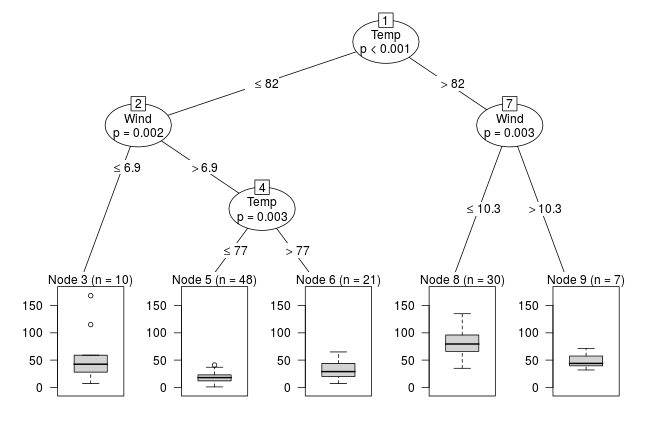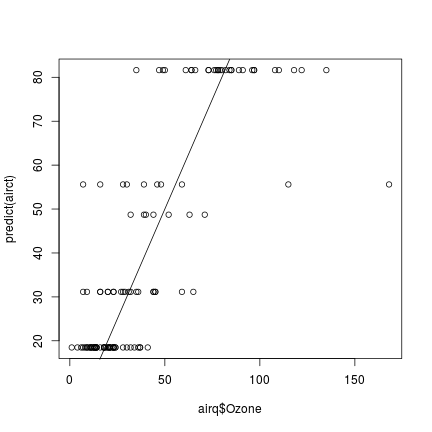First, install and fire-up R on your computer. Within R, one needs to install the party package by typing

install.packages("partykit")


and hitting the ENTER key. Once the package is installed, you can load it using

library("partykit")

## Loading required package: grid

## Loading required package: libcoin

## Loading required package: mvtnorm

## Loading required package: rpart


Now all party functions are ready to be used, for example the ctree() function for fitting a regression tree to the Ozone data (after removing observations with missing response):

### regression
airq <- subset(airquality, !is.na(Ozone))
airct <- ctree(Ozone ~ ., data = airq,
control = ctree_control(maxsurrogate = 3))
airct

##
## Model formula:
## Ozone ~ Solar.R + Wind + Temp + Month + Day
##
## Fitted party:
##  root
## |    Temp <= 82
## |   |    Wind <= 6.9: 55.600 (n = 10, err = 21946.4)
## |   |    Wind > 6.9
## |   |   |    Temp <= 77: 18.479 (n = 48, err = 3956.0)
## |   |   |    Temp > 77: 31.143 (n = 21, err = 4620.6)
## |    Temp > 82
## |   |    Wind <= 10.3: 81.633 (n = 30, err = 15119.0)
## |   |    Wind > 10.3: 48.714 (n = 7, err = 1183.4)
##
## Number of inner nodes:    4
## Number of terminal nodes: 5


The tree is represented by an object called airct which can be plotted

plot(airct)or used for computing predictions

summary(predict(airct))

##    Min. 1st Qu.  Median    Mean 3rd Qu.    Max.
##   18.48   18.48   31.14   42.13   81.63   81.63


which can be compared to the actual response values:

plot(airq\$Ozone, predict(airct))
abline(a = 0, b = 1)